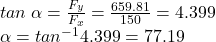## Two teenagers are pulling on ropes attached to a tree. The angle between the ropes is 30.0°30.0° . David pulls with a force of 400.0 N and S

Question

Two teenagers are pulling on ropes attached to a tree. The angle between the ropes is 30.0°30.0° . David pulls with a force of 400.0 N and Stephanie pulls with a force of 300.0 N. (a) Find the component form of the net force. (b) Find the magnitude of the resultant (net) force on the tree and the angle it makes with David’s rope.

in progress 0
2 months 2021-07-26T17:39:32+00:00 1 Answers 1 views 0

The magnitude of the resultant force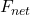, is 676.643 N

The angle it makes with David;s rope is 77.19°

Explanation:

Here we have

David’s force F₁ = 400 N and direction of F₁ = horizontal θ₁ = 0

Stephanie’s force F₂ = 300.0 N direction of F₂ = θ₂ = 30°

The angle between the ropes θ = 30°

Therefore we resolve the forces applied by the two teenagers as follows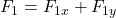F₁ₓ = F₁×cos (0) = F₁ = 400.0 N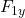=  F₁×sin (0) = 0 N

Also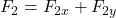F₂ₓ = F₂×cos (θ₂) = 300 ×cos (30)= 259.81 N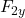=  F₂×sin (θ₂) =  300 ×sin (30)= 150 N

Therefore, the combined force of both teenagers is;

∑Fₓ = F₁ₓ + F₂ₓ = 400.0 N + 259.81 N = 659.81 N and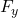=+= 0 N + 150 N =150 N

The resultant force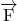is given by=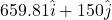The magnitude of the resultant force is,;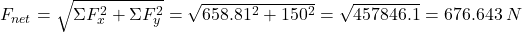The angle it makes with David’s rope is